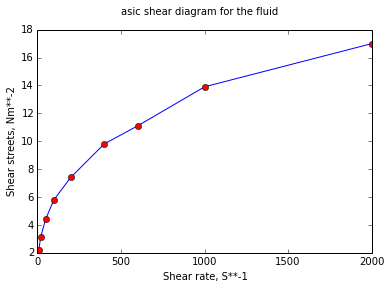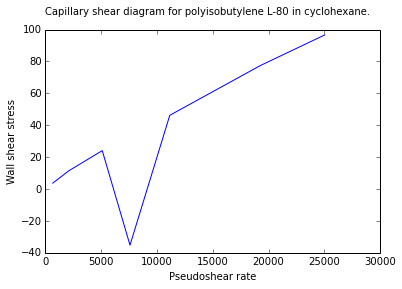# Chapter 15 : Non newtonian phenomena¶

### Example 15.1 - Page No :760¶

In :
%matplotlib inline

import math
from numpy import *
from matplotlib.pyplot import *

# Variables
# given
r = array([10, 20, 50, 100, 200, 400, 600, 1000, 2000])
tau = array([2.2, 3.1 ,4.4, 5.8, 7.4, 9.8, 11.1, 13.9, 17.0])

# Calculation and Results
#tau = tau*(10**-4);
plot(r,tau);
plot(r,tau,'ro');
suptitle("asic shear diagram for the fluid")
xlabel("Shear rate, S**-1 ")
ylabel("Shear streets, Nm**-2 ")

# the data falls nearly on a straight line
# from the graph the slope and the intercept are
slope = 0.3841;
intercept = 9.17046;
# from the relation tau = K*(-r)**n;
K = math.exp(intercept);
n = slope
print "K = ",K
print "n = ",n
print " The fluid is pseudo plastic, since the slope is less than 1 "

Populating the interactive namespace from numpy and matplotlib
K =  9609.04383369
n =  0.3841
The fluid is pseudo plastic, since the slope is less than 1

WARNING: pylab import has clobbered these variables: ['draw_if_interactive']
%pylab --no-import-all prevents importing * from pylab and numpy### Example 15.2 - Page No :774¶

In :
# Variables
a = array([651, 1361, 2086, 5089, 7575, 11140, 19270, 25030])
tau = array([3.71, 7.49, 11.41, 24.08, -35.21, 46.25, 77.50, 96.68])

# from the graph
betao = -4.3790154;
beta1 = 0.8851;

# Calculations
K = math.exp(betao);
n = beta1;
plot(a,tau);
suptitle("Capillary shear diagram for polyisobutylene L-80 in cyclohexane.")
xlabel("Pseudoshear rate")
ylabel("Wall shear stress ")

# Results
print " The final rheological model_ is  tauw  =  %f*8*Uz,avg/do)**%f"%(K,n);

 The final rheological model_ is  tauw  =  0.012538*8*Uz,avg/do)**0.885100### Example 15.3 - Page No :774¶

In :
# Variables
# from Example  15.2
n = 0.8851;
K = 0.01254;
n = n;

# Calculations
K = K/((3*n+1)/(4*n));

# Results
print "n = ",n
print "K  =  %f N/m**2"%(K);

n =  0.8851
K  =  0.012146 N/m**2


### Example 15.4 - Page No :775¶

In :
# Variables
a = array([10, 20, 50, 100, 200, 400, 600, 1000, 2000])
tau = array([2.24, 3.10, 4.35, 5.77, 7.50, 9.13, 11.0, 13.52, 16.40])
tau = tau*10**-4;
betao = 8.96694;
beta1 = 0.48452520;
beta2 = 0.010923041;

# Calculations
# such a plot suggests a second order polynomila  of the type y = betao+beta1*x+beta2*x**2;
# where y = ln(tauw) and x = ln(8*Uz,avg/do) = ln(a);
# from the graph
n = beta1+2.*beta2*a;
phiw = ((3.*n+1.)/(4.*n))*(a);
mu = tau/phiw;

# Results

print " 8*Uz,avg/do     n         ((3*n+1)/4*n)    phiw            mu"
for i in range(9):
print " %6.0f       %8.4f      %8.4f      %8.4f       %6.6f"%(a[i],n[i],3*n[i]+1/4*n[i],phiw[i],mu[i])


 8*Uz,avg/do     n         ((3*n+1)/4*n)    phiw            mu